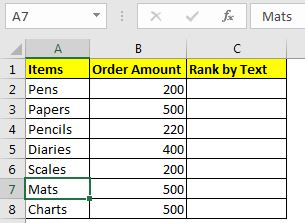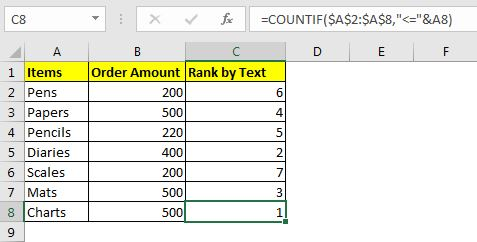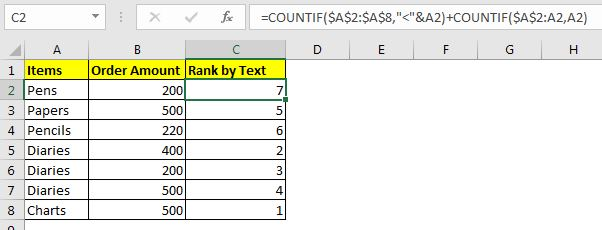# Excel Formula to Sort Text

Earlier, we learned about the sorting of numeric values in Excel. In this article, we will learn how to sort text values using an Excel formula. It will be kind of ranking, based on ASCII number of characters in the text.

Here's the generic formula to sort text in a range

 =COUNTIF(text_range,"=<"&first_text_ref)

text_range: The range that contains the text values.

first_text_ref: The reference of the first cell in the text_range.

Let's see this formula in action:

## Example: Rank and Sort data based on the item namesHere again, we have that list of stationery items. All items are unique. This time we want to sort it based on the names of the item.

The RANK function does not work with texts, hence we can't use it. We will use the COUNTIF function, as you can see in the general formula above.

If you are following this example, write the above formula in C2 and drag it down.

 =COUNTIF(\$A\$2:\$A\$8,"<="&A2)You have your items ranked as per their name.

Note: The text values must be unique, otherwise there will be ties.

How does it work?

So, you must be wondering how we can use <= with text in Excel with COUNTIF function? Well, Excel compares the ASCII values of two text values.

In the formula above, Excel checks how many values in range A2:A8 are less than or equal to text in A8. It returns 6. 5 values are less than it and itself is equal to it (English!!!!), making it 6. If you only keep less than sign, then the ranking will start from 0 to n-1.

As you copy this formula in below cells, the formula sorts out all the rankings accurately, as long as the list is unique. If you have duplicate text values, then we have to make a minor change to this formula.

### Ranking for sorting of text with duplicates

 =COUNTIF(\$A\$2:\$A\$8,"<"&A2)+COUNTIF(\$A\$2:A2,A2)

Here, we are having removed = sign from the formula and added the running count of text values to it. Now the ranking/sorting starts from 0 and we add each occurrence of each text to the ranking. This solves the duplicate problem.Now you can formulas to get sorted results. INDEX and MATCH are the functions that work on indexes.

Related Articles:

Sort Numeric Values with Excel RANK Function | To sort numeric values in as a helping column, we use RANK and COUNTIF function.

Sort numbers using Excel SMALL function | To sort number ascendingly or descendingly with excel formulas we use the SMALL function along with the ROW. This excel sort formula is accurate and to the point.

Expanding References in Excel | These ranges expand when copied down or to right. They help in running calculations in Excel.

How to do Running Count of Occurance in Excel | Use expanding ranges to count values as per they occur.

Popular Articles:

50 Excel Shortcuts to Increase Your Productivity | Get faster at your task. These 50 shortcuts will make you work even faster on Excel.

The VLOOKUP Function in Excel | This is one of the most used and popular functions of excel that is used to lookup value from different ranges and sheets.

COUNTIF in Excel 2016 | Count values with conditions using this amazing function. You don't need filter your data to count specific value. Countif function is essential to prepare your dashboard.

How to Use the SUMIF Function in Excel | This is another dashboard essential function. This helps you sum up values on specific conditions.

Terms and Conditions of use

The applications/code on this site are distributed as is and without warranties or liability. In no event shall the owner of the copyrights, or the authors of the applications/code be liable for any loss of profit, any problems or any damage resulting from the use or evaluation of the applications/code.